Parents can use S Chand Class 9 Maths Solutions ICSE Chapter 16 Area of Plane Figures Ex 16(D) to provide additional support to their children.

## S Chand Class 9 ICSE Maths Solutions Chapter 16 Area of Plane Figures Ex 16(D)

Question 1.
Find the area of the trapezium if the :
(i) Parallel sides are 3 cm and 6 cm and perp. distance between them is 10 cm.
(ii) Parallel sides are 25 m and 33 m and perp. distance between them is 20 m.
Solution:
(i) Length of parallel sides are
3 cm and 6 cm
and perpendicular distance (h) between them = 10 cm∴ Area = $$\frac { 1 }{ 2 }$$ (Sum of parallel sides) × height
= $$\frac { 1 }{ 2 }$$ (6 + 3) × 10 = 9 × 5 = 45 cm2

(ii) Parallel sides are 25 m and 33 m
and perpendicular distance (h) = 20 m
∴ Area = $$\frac { 1 }{ 2 }$$ (sum of parallel sides × height)
= $$\frac { 1 }{ 2 }$$ (25 + 33) × 20 = 58 × 10 = 582 m2

Question 2.
The area of a trapezium is 240 m2 and the sum of the parallel sides is 48 m. Find the height.
Solution:
Area of trapezium = 240 m2
Sum of parallel sides = 48 m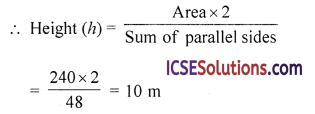Question 3.
The parallel sides of a trapezium are 4.36 cm and 3.18 cm and area is 18.85 cm2. Find the distance between the parallel sides.
Solution:
Parallel sides of trapezium are 4.36 cm and 3.18 cm
Area = 18.85 cm2
∴ Distance between the parallel sides (h)Question 4.
The area of a trapezium is 475 cm2 and the height is 19 cm. Find its two parallel sides if one side is 4 cm greater than the other.
Solution:
Area of trapezium = 475 cm2
Height (h) = 19 cm
∴ Sum of two parallel sides = $$\frac{\text { Area } \times 2}{\text { Height }}$$
= $$\frac{475 \times 2}{19}$$ = 50 cm
Let one of the two parallel sides = x
Then second side = x + 4
∴ x + x + 4 = 50 ⇒ 2x = 50 – 4 = 46
x = $$\frac { 46 }{ 2 }$$ = 23
∴ First side = 23 cm
and second side = 23 + 4 = 27 cm

Question 5.
The parallel sides of a trapezium are in the ratio 2 : 5 and the distance between the parallel sides is 10 cm. If the area of the trapezium is 350 cm2, find the lengths of its parallel sides.
Solution:
Ratio in parallel sides = 2 : 5
Distance between them (h) = 10 cm
and area = 350 cm2
∴ Sum of parallel sides = $$\frac{\text { Area } \times 2}{\text { Height }}$$
= $$\frac{350 \times 2}{10}$$ = 70 cm
Sum of ratio = 2 + 5 = 7
∴ First side = $$\frac{70 \times 2}{7}$$ = 20 cm
and second side = $$\frac{70 \times 5}{7}$$ = 50 cm

Question 6.
In the figure, AD = BC = 5 cm, AB = 7 cm. The parallel sides AB, DC are 4 cm apart. DC = x cm. Find x and the area of the trapezium ABCD.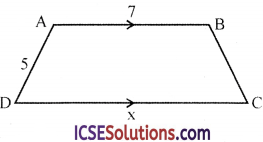Solution:
In the figure, ABCD is a trapezium in which
AD = BC = 5 cm
AB = 7 cm, DC = x cm
and distance between the parallel sides = 4 cm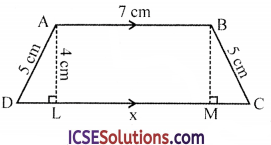Draw AL and BM ⊥s on DC
AL = BM = 4 cm
AB = LM = 4 cm
∵ AD = BC = 7 cm
∴ ABCD is an isosceles trapezium
Now in right △ALD
AD2 = AL2 + LD2 (Pythagoras Theorem)
(5)2 = (4)2 + LD2
⇒ 25 = 16 + LD2
LD2 = 25 – 16 = 9 = (3)2
∴ x = LD = 3 cm
∴ DC = AB + 2 LD
= 7 + 2 × 3 = 7 + 6 = 13 cm
Now area of trapezium = $$\frac { 1 }{ 2 }$$ (sum of parallel sides) × height
= $$\frac { 1 }{ 2 }$$ (7 + 13) × 4
= $$\frac { 1 }{ 2 }$$ × 20 × 4 = 40 cm2
∴ x = 13 and area = 40 cm2

Question 7.
The parallel sides of a trapezium are 7.5 cm, 3.9 cm, and the other sides are each 2.6 cm. Find its area.
Solution:
In trapezium ABCD
AB = 7.5 cm, CD = 3.9 cm
AD = BC = 2.6 cm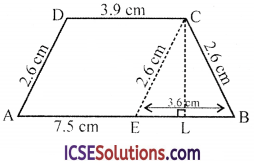Draw CE || DA, CL ⊥ AB
∴ CE = 2.6 cm and AE = DC = 3.9 cm
∴ EB = AB – AE = 7.5 – 3.9 = 3.6 cm
In an isosceles △CEB, CL ⊥ EB
Which bisects EB at L
∴ EL = $$\frac { 1 }{ 2 }$$ EB = $$\frac { 1 }{ 2 }$$ × 3.6 = 1.8 cm
Now in right △CEL
CE2 = CL2 + EL2 (Pythagoras Theorem)
⇒ (2.6)2 = CL2 + (1.8)2
⇒ 6.76 = CL2 + 3.24
⇒ CL2 = 6.76 – 3.24 = 3.52
∴ CL = $$\sqrt{3.52}$$ = 1.876
Now area of trapezium = $$\frac { 1 }{ 2 }$$ (sum of parallel sides) × height
= $$\frac { 1 }{ 2 }$$ (7.5+3.9) × 1.876
= $$\frac { 1 }{ 2 }$$ × 11.4 × 1.186 = 5.7 × 1.876 cm2
=10.6932 cm2
= 10.7 cm2Question 8.
The given figure shows that cross section of a concrete structure with the measurments, as given. Calculate the area of the cross section.Solution:
In the figure, produce DE to meet AB at G Now we have one trapezium GBCD and one rectangle AGEF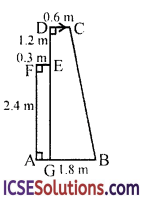Now GB = AB – AG = AB – FE = 1.8 – 0.3 = 1.5 cm
and DG = DE + EG = DE + FA = 1.2 + 2.4 = 3.6 cm
Now area of rectangle AGEF = l × b = 2.4 × 0.3 = 0.72 cm2
and area of trapezium GBCD = $$\frac { 1 }{ 2 }$$ (sum of parallel sides) × height
= $$\frac { 1 }{ 2 }$$ (GB + DC) × DG
= $$\frac { 1 }{ 2 }$$ (1.5 + 0.6) × 3.6 cm2
= $$\frac { 1 }{ 2 }$$ × 2.1 × 3.6 = 2.1 × 1.8 cm2
= 3.78 cm2
Area of the whole figure = 0.72 + 3.78
= 4.50 cm2 = 4.5 cm2

Question 9.
In the figure, find
(i) AB
(ii) Area of the trapezium ABCD.Solution:
(i) In the figure, draw CE || AB meeting AD in E, then
AE = BC = 2 and DE = AD – EA
=8 – 2 = 6 cm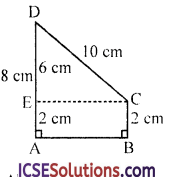In right △ECD
CD2 = D2 + EC2 (Pythagoras Theorem)
(10)2 = (6)2 + E2
100 = 36 + E2
⇒ EC2 = 100 – 36 = 64 = (8)2
∴ EC = 8 cm or AB = EC = 8 cm

(ii) Now area of trapezium ABCD
= $$\frac { 1 }{ 2 }$$ (sum of parallel sides) × height
= $$\frac { 1 }{ 2 }$$ (AD + BC) × AB
= $$\frac { 1 }{ 2 }$$ (8 + 2) × 8 = $$\frac { 1 }{ 2 }$$ × 10 × 8 cm2 = 40 cm)2

Question 10.
The cross-section of a tunnel perpendicular to its length is a trapezium ABCD as shown in the figure. AM = BN ; AB = 4.4 m; CD = 3 m. The height of the tunnel is 2.4 m. The tunnel is 50 m long.Calculate :
(i) the cost of painting the internal surface of the tunnel (excluding the floor) at the rate of Rs. 5 per m2.
(ii) the cost of paving the floor at the rate of Rs. 18 per m2.
Solution:
In the figure, ABCD is a trapezium in which AM = BN, AB = 4.4 m, CD = 3 m and height of the tunnel = 2.4 m
Length of tunnel = 50 m
∵ DM and CN are perpendiculars on AB and
AM = NB = $$\frac { 1 }{ 2 }$$ (AB – DC)
= $$\frac { 1 }{ 2 }$$ (4.4 – 3.0) m
= $$\frac { 1 }{ 2 }$$ (1.4) = 0.7 m
AD2 = DM2 + AM2 = (2.4)2 + (0.7)2
= 5.76 + 0.49 = 6.25 = (2.5)2
∴ AD = CB = 2.5 m

(i) Now area of the interval surface of the tunnel (excluding floor) = Perimeter of the face × Length
= (3 + 2.5 + 2.5) × 50 m2
= 8 × 50 = 400 m2
Rate of painting = Rs. 5 per m2
Total cost = Rs. 400 × 5 = Rs. 2000

(ii) Area of floor = 4.4 × 50 = 220 m2
Rate of paving = Rs. 18 per m2
∴ Total cost of paving the floor = Rs. 18 × 220 = Rs. 3960

Question 11.
A trapezium with its parallel sides in the ratio 11 : 3 is cut off from a rectangle whose sides measure 98 cm and 12 cm. The area of the trapezium is $$\frac { 3 }{ 7 }$$ of the area of the rectangle. Find the lengths of the parallel sides of the trapezium, when its height is equal to the smaller side of the rectangle.
Solution:
Sides of rectangle are 98 cm and 12 cm
∴ Area = l × b = 98 × 12 cm2 = 1176 cm2
∴ Area of trapezium = $$\frac { 3 }{ 7 }$$ of 1176 = 504 cm2
Height of trapezium (h) = smaller side of rectangle = 12 cm
∴ Sum of parallel sides = $$\frac{\text { Area } \times 2}{\text { Height }}$$ = $$\frac{504 \times 2}{12}$$ cm
=42 × 2 = 84 cm
Ratio of the parallel sides = 11 : 3
Sum of ratios = 11 + 3 = 14
∴ First side = $$\frac{84 \times 11}{14}$$ = 66 cm
and second side = $$\frac{84 \times 3}{14}$$ = 18 cmQuestion 12.
The cross-section of a canal is in the shape of a trapezium. If the canal is 12 m wide at the top and 8 m wide at the bottom and the area of its cross-section is 84 m2, determine its depth.
Solution:
Area of cross-section = 84 m2
Sides are 12 m and 8 m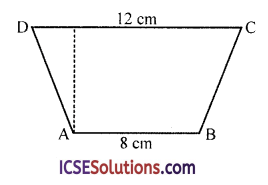Let h be its depth, then
h = $$\frac{\text { Area } \times 2}{\text { Sum of parallel sides }}$$
= $$\frac{84 \times 2}{(12+8)}$$ = $$\frac{84 \times 2}{20}$$ = $$\frac { 168 }{ 20 }$$ m = 84 m
∴ Depth of the canal = 8.4 m